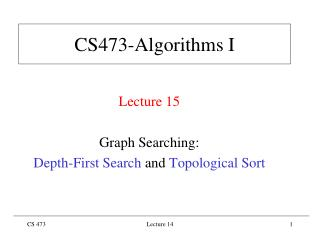# CS473-Algorithms I - PowerPoint PPT PresentationDownload PresentationCS473-Algorithms I

CS473-Algorithms I
Download Presentation## CS473-Algorithms I

- - - - - - - - - - - - - - - - - - - - - - - - - - - E N D - - - - - - - - - - - - - - - - - - - - - - - - - - -
##### Presentation Transcript

1. CS473-Algorithms I Lecture 15 Graph Searching: Depth-First Search and Topological Sort Lecture 14

2. Depth-First Search • Graph G(V,E) directed or undirected • Adjacency list representation • Goal: Systematically explore every vertex and every edge • Idea: search deeper whenever possible • Using a LIFO queue (Stack; FIFO queue used in BFS) Lecture 14

3. Depth-First Search • Maintains several fields for each vV • Like BFS, colors the vertices to indicate their states. Each vertex is • Initially white, • grayed when discovered, • blackened when finished • Like BFS, records discovery of a white v during scanning Adj[u] by [v] u Lecture 14

4. Depth-First Search • Unlike BFS, predecessor graph G produced by DFS forms spanning forest • G(V,E) where E{([v],v): vV and[v] NIL} • G depth-first forest (DFF) is composed of disjoint depth-first trees (DFTs) Lecture 14

5. Depth-First Search • DFS also timestamps each vertex with two timestamps • d[v]: records when v is first discovered and grayed • f[v]: records when v is finished and blackened • Since there is only one discovery event and finishing event for each vertex we have 1 d[v]  f[v] 2|V| Time axis for the color of a vertex d[v] f[v] 2|V| 1 2 Lecture 14

6. Depth-First Search DFS-VISIT(G, u) color[u] gray d[u] time time1 for eachv Adj[u] do if color[v] whitethen [v]  u DFS-VISIT(G, v) color[u] black f[u] time time1 DFS(G) for eachuV do color[u] white [u]  NIL time 0 for eachuV do if color[u] whitethen DFS-VISIT(G, u) Lecture 14

7. Depth-First Search • Running time: (VE) • Initialization loop in DFS : (V) • Main loop in DFS: (V) exclusive of time to execute calls to DFS-VISIT • DFS-VISIT is called exactly once for each vV since • DFS-VISIT is invoked only on white vertices and • DFS-VISIT(G, u) immediately colors u as gray • For loop of DFS-VISIT(G, u) is executed |Adj[u]| time • Since  |Adj[u]|  E, total cost of executing loop of DFS-VISIT is (E) Lecture 14

8. Depth-First Search: Example Lecture 14

9. Depth-First Search: Example Lecture 14

10. Depth-First Search: Example Lecture 14

11. Depth-First Search: Example Lecture 14

12. Depth-First Search: Example Lecture 14

13. Depth-First Search: Example Lecture 14

14. Depth-First Search: Example Lecture 14

15. Depth-First Search: Example Lecture 14

16. Depth-First Search: Example Lecture 14

17. Depth-First Search: Example Lecture 14

18. Depth-First Search: Example Lecture 14

19. Depth-First Search: Example Lecture 14

20. Depth-First Search: Example Lecture 14

21. Depth-First Search: Example Lecture 14

22. Depth-First Search: Example Lecture 14

23. Depth-First Search: Example Lecture 14

24. Depth-First Search: Example Lecture 14

25. Depth-First Search: Example Lecture 14

26. Depth-First Search: Example Lecture 14

27. Depth-First Search: Example Lecture 14

28. Depth-First Search: Example Lecture 14

29. Depth-First Search: Example Lecture 14

30. Depth-First Search: Example Lecture 14

31. Depth-First Search: Example Lecture 14

32. Depth-First Search: Example DFS(G) terminated Depth-first forest (DFF) Lecture 14